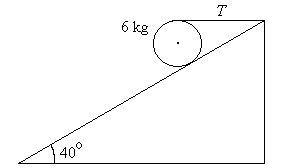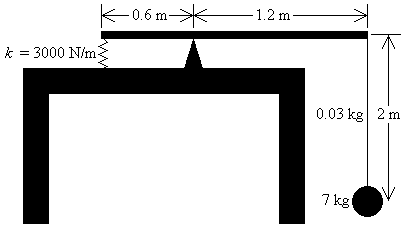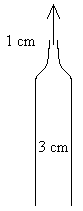First Name: ___________________ Last Name: ____________________ Section: _________

November 20, 1998 Physics 207

Exam 3

Print your name and section clearly on all five pages. (If you do not know your section number, write your TAs name.) Show all work in the space immediately below each problem. Your final answer must be placed in the box provided. Problems will be graded on reasoning and intermediate steps as well as on the final answer. Be sure to include units wherever necessary, and the direction of vectors. Each problem is worth 25 points. In doing the problems, try to be neat. Check your answers to see that they have the correct dimensions (units) and are the right order of magnitudes. You are allowed one 8½ x 11" sheet of notes and no other references. The exam lasts exactly 50 minutes.

(Do not write below)

SCORE:

Problem 1: __________

Problem 2: __________

Problem 3: __________

Problem 4: __________

TOTAL: ___________

First Name: ___________________ Last Name: ____________________ Section: _________

1. A uniform cylinder with a mass of 6 kg is held at rest by a horizontal wire on a plane inclined at an angle of 40° as shown.a. What is the tension T in the wire? (13 pts.)

b. If the wire breaks, what is the acceleration of the center of mass of the cylinder? (12 pts.)

First Name: ___________________ Last Name: ____________________ Section: _________

2. A bowling ball with a mass of 7 kg is suspended in static equilibrium by a wire 2 m long with a mass of 0.03 kg from one end of a massless, horizontal beam 1.8 m long. The beam rests on a fulcrum 0.6 m from the opposite end which is attached to the floor by a spring with k = 3000 N/m as shown.a. If the bowling ball is displaced horizontally by a small distance and released, what is the period of the resulting oscillation? (6 pts.)

b. If the bowling ball is displaced vertically by a small distance and released, what is the period of the resulting oscillation? (7 pts.)

c. If the wire is struck at one end with a hammer, how long does it take for the resulting wave to travel to the other end of the wire? (6 pts.)

d. If the wire has a Youngs modulus of 2 x 1011 N/m2 and a diameter of 1 mm, how much does it stretch under the weight of the bowling ball? (6 pts.)

First Name: ___________________ Last Name: ____________________ Section: _________

3. A 50-kg satellite is in a circular orbit around the earth (RE = 6370 km) at a height of 2000 km above the surface of the earth.

1. What is the orbital speed of the satellite? (7pts.)

2.

3. What is the orbital period of the satellite? (6 pts.)

c. What is the angular momentum of the satellite? (6 pts.)

d. If the thrusters are fired so that the satellite is brought momentarily to rest, what kinetic energy does the satellite have just before it hits the earth? (6 pts.)

First Name: ___________________ Last Name: ____________________ Section: _________

4. A fountain at sea level consists of a 3-cm-diameter pipe with a 1-cm-diameter nozzle that sprays water straight up to a height of 30 m.a. What is the velocity of the water as it leaves the nozzle? (5 pts.)

b. What volume of water is ejected per second? (5 pts.)

c. What is the velocity of the water in the pipe? (5 pts.)

d. What is the pressure of the water in the pipe? (5 pts.)

e. How many watts must the water pump supply? (5 pts.)